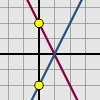#### You may also like### Enclosing Squares

Can you find sets of sloping lines that enclose a square?### Diamond Collector

Collect as many diamonds as you can by drawing three straight lines.### Reflecting Lines

Investigate what happens to the equations of different lines when you reflect them in one of the axes. Try to predict what will happen. Explain your findings.

# Parallel Lines

##### Age 11 to 14Challenge Level

Georgina from St. George's School correctly identified that it is the value of the number before the $x$ (the coefficient) that is important in determining how steep the line is, and how the number $x$ is multiplied or divided by relates to how it crosses the squares.

The steeper the line is, the larger the number before $x$ becomes. You can work this number out by counting how many squares up the line travels for every one across. The number up is the number before $x$.

The shallower the line, the larger the number x is divided by. You can work out this number by counting how many squares the line goes across for every one up.

Put the 2 together and you end up with an equation like the one below

$\frac{2x}{3}$ Simple!

Josh from Melbourn Village College pointed out that for two lines to be parallel, the formulae had to be the same except for the constant value added or subtracted and that this related to the distance of one line above/below the other. He also correctly came up with the rule for the formulae of vertical and horizontal lines.

When the red dot of the green line is on 0 to make the red line parallel below, the formula has to be the same but with minus however many squares there is difference.

If the red line is on 0 and the green is above then formula is the same but the green lines formula has plus the difference between them.

If both lines are horizontal the the formula for both is simple - its one number of how much the difference is from 0 e.g. $y=2$, $x=-7$

Both Daniel from AMVC and Beth, again from Melbourn Village College, correctly quoted the general equation for a straight line $y=mx+c$ and explained that providing the values of $m$ in the equations of two lines were the same, they would be parallel.

Daniel:

All straight lines can be expressed with the equation $y=mx+c$, where $m$ is the gradient (how steep the line is). This means that as long as the $m$ for both equations are equal the lines will be parallel.

Beth:

The equations of parallel lines are the same, except for the value of the constant (the value on the end of an equation). So, a line's equation will follow this structure: $y = mx$, but its parallel would follow this structure: $y = mx + c$.

Emma and Chloe from The Mount School noticed how the position of a line affects the equation:

When a line has a positive gradient, the equation never includes negative values of $x$.

When the line goes through zero there are no $x$ + or - somethings.

If the line does not go through zero then it will be $x$ + or - something else on the end of the equation.

The equations of parallel lines always have the same gradient but cross the $y$ axis at different points.

Boyang from Mountfields Lodge School added:

If the lines are parallel then it is always a $y = ax + b$ equation.

The $ax$ part is the same on both equations when the lines are parallel.